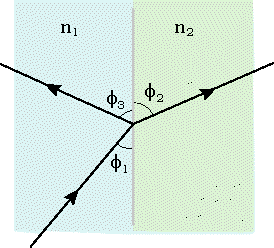# Finding the Velocity of Light through an Unknown substance

## Homework StatementIn the above figure, a ray of light is incident upon the interface between two media having indices of refraction n1 = 1.2 and n2, which is unknown. The known angles are f2 = 60° and f3 = 29.9°. (Note that the angles in the diagram may not be accurate.) What is the speed of light in the second medium?

## Homework Equations

Snell's Law n$_{1}$sin θ$_{1}$ = n$_{2}$sin θ$_{2}$

And the velocity of light equation:

n = $\frac{c}{v_{n}}$

## The Attempt at a Solution

Since the Angles given aren't the "true" angles that are used in Snell's Law

I took each angle and subtract them from 90° to get θ$_{2}$ = 30° and θ$_{3}$ = 60.1°

From there, I calculated that θ$_{1}$ = 29.9°

I took the variables n$_{1}$ = 1.2 and θ$_{1}$ & θ$_{2}$ and plugged them into the Snell's Formula to get n$_{2}$ = 1.196 as the index of refraction of the unknown substance.

I then plugged that calculation into the velocity formula to get 2.5 x 10$^{8}$, but I get that as the wrong answer.

I don't know where I went wrong with this. Thank you for your help!

## Answers and Replies

TSny
Homework Helper
Gold Member
I took each angle and subtract them from 90° to get θ$_{2}$ = 30° and θ$_{3}$ = 60.1°

From there, I calculated that θ$_{1}$ = 29.9°

What does the "law of reflection" tell you about θ1 and θ3.

The Law's of reflection would state that θ$_{1}$ = θ$_{3}$. I then got the angle as 60.1 degrees. I was looking at the picture incorrectly and used the wrong angles that were required for snell's Law. I got it now!

Thanks TSny!

Last edited: